# Merging Call and Put Payoff Calculations

This is part 2 of the Option Payoff Excel Tutorial, where we are building a calculator that will compute option strategy profit or loss and draw payoff diagrams. In the first part we have explained the payoff formulas and created a simple spreadsheet that calculates profit or loss for a single call and put option: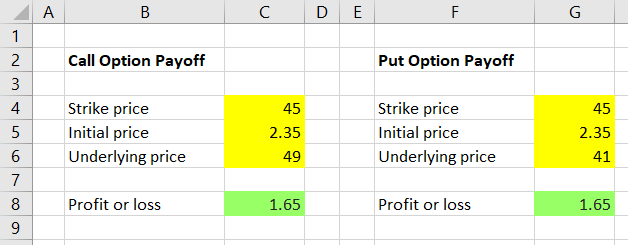Now we are going to merge the two calculations into one, which will make our calculator more user-friendly and allow us to make further improvements.

The idea is to have one calculation working for both calls and puts, with the option type (call or put) as another user input. Let's place this new input in cell C3: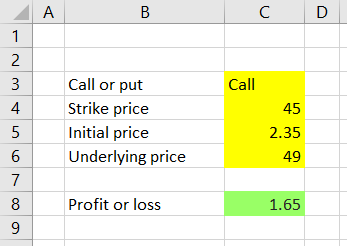At the moment cell C8 always calculates call payoff, using the formula which we have created in the previous part:

``=MAX(C6-C4,0)-C5``

We must change this formula to

• calculate call payoff only if the value of cell C3 is "Call" and
• calculate put payoff if the value of cell C3 is something else (for example, "Put").

## Excel IF Function

We can do this easily using Excel IF function, which takes three parameters and looks like this:

``=IF(condition, result if condition is true, result if condition is false)``

In our case:

``=IF(cell C3 is "Call", call option payoff, put option payoff)``

Using the correct Excel syntax, the formula in cell C8 is:

``=IF(C3="Call",MAX(C6-C4,0)-C5,MAX(C4-C6,0)-C5)``

... where:

• `C3="Call"` tests whether cell C3 content is "Call"
• `MAX(C6-C4,0)-C5` calculates call option profit or loss (the previous formula in cell C8)
• `MAX(C4-C6,0)-C5` calculates put option profit or loss (the same formula as in cell G8, only with the input references changed from G4, G5, G6 to C4, C5, C6)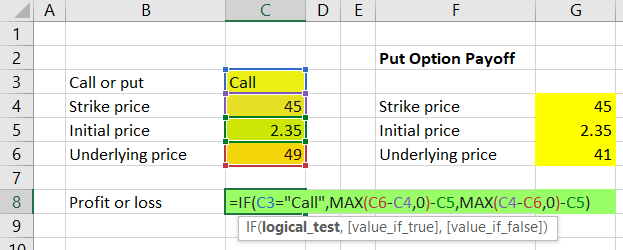Now cell C8 will show call or put option profit or loss, based on the inputs in cells C3-C6.

We can delete the put option payoff calculation in columns F-G, because we no longer need it.

That said, there are still two ways we can improve our merged calculation.

## Putting the IF inside the MAX

You can see that the formulas for call and put option payoff (the second and third parameter of our IF function) are very similar. The only difference is the `C6-C4` for calls vs. `C4-C6` for puts; the rest is exactly the same. Therefore, we are repeating ourselves a little having two almost identical MAX functions inside a very long IF function.

We can simplify the formula and only put the part that is different (`C6-C4` or `C4-C6`) inside the IF, leaving the common parts (the rest of the MAX minus initial cost) outside the IF. Rather than putting two MAXes inside one long IF, we will put a much smaller IF inside just one MAX.

The formula in cell C8 becomes:

``=MAX(IF(C3="Call",C6-C4,C4-C6),0)-C5``

The result of this simplified formula will always be the same as the original one, which is still valid and correct. It is just a matter of better design, which becomes more important when you work with more complex calculations. More often than not, shorter and simpler formulas are better than long ones.

## Fixing the Call/Put Input

The other improvement is much more important. At the moment, the IF function checks whether the option type input in cell C3 is "Call" and uses the call option payoff formula if it is, but uses the put option formula in all other cases.

As a result, when a user enters something like "C" or "call option" in cell C3, the formula in cell C8 will not recognize it as a call option (because it is looking for the exact word "Call"). It will return put option payoff, even when the user wanted a call.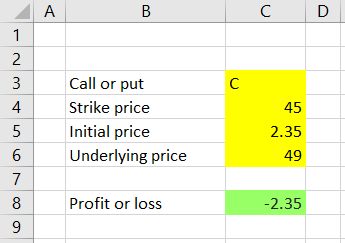In other words, our existing implementation expects the user to be very precise and to know the exact format of the input. This can lead to confusion and errors. It is bad design and should be fixed.

We can try to fix it by changing the IF condition in cell C8 to also consider the values "C" and "call option", using the OR Excel function:

``=MAX(IF(OR(C3="Call",C3="C",C3="call option"),C6-C4,C4-C6),0)-C5``

But a much better solution is to force the users to only select Call or Put in cell C3 in the first place, instead of letting them type the words. We can do this with dropdown box: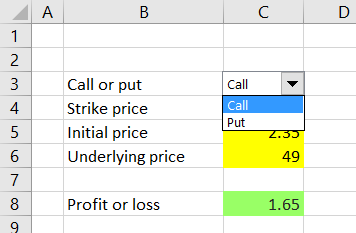Our combo box with have two options to choose from – "Call" and "Put". Type these words in cells F3 and F4, respectively.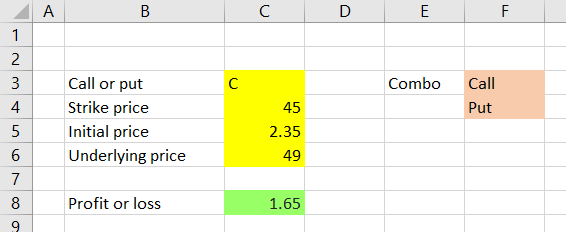Now we can add the actual combo box in cell C3.

Some people may not be familiar with combo boxes and how to add them to spreadsheets, but what may seem like advanced Excel is actually quite simple yet often very useful. If you already know how to add combos, you can skip the following few paragraphs and screenshots.

You can add a combo box by clicking the Developer tab in your Excel main menu, then the Insert icon and than the small combo box icon, as shown in the screenshot below (it is from Excel 2016 and may look a little different in other versions).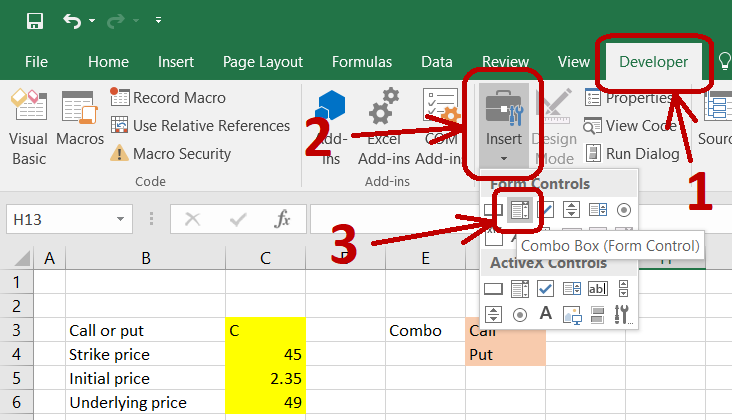If you can't find the Developer tab in your main menu (it is usually the last tab at the right end), you must first enable it under File / Options / Customize Ribbon. If you are not sure how to do this in your Excel version, google "how to enable developer tab in excel [your version]".

When you finally click the combo box icon, your mouse pointer will change to a little plus, which means you should draw a small rectangle with it to show where you want the combo box to be located in your spreadsheet (don't worry, you can always move or resize it later). We will draw our rectangle over cell C3.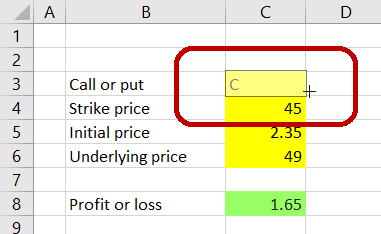The result is a new empty combo box: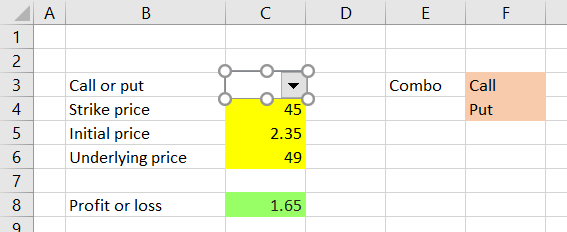We want our combo box to show the "Call" or "Put" text from cells F3 and F4, and based on the user's selection change the value of cell C3 (which will be hidden behind the combo box, but still there and working as a standard cell).

Point your mouse to the combo box and on a right-click a menu will appear. Click on "Format Control" at the bottom.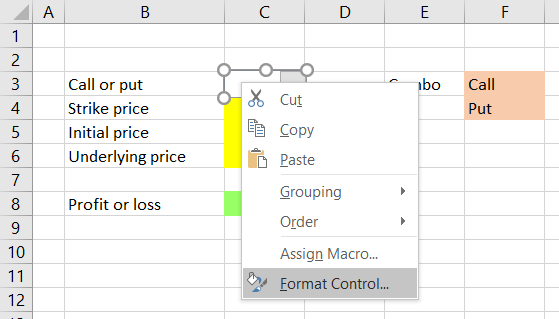A new window will appear, where we can set our cell references:

• "Input range" is F3-F4, where our two text options are stored.
• "Cell link" is the cell which will store the selection result – C3.
• "Drop down lines" is how high you want the dropdown box to be when clicked and displaying the options – in our case just 2 lines, one for "Call" and the other for "Put".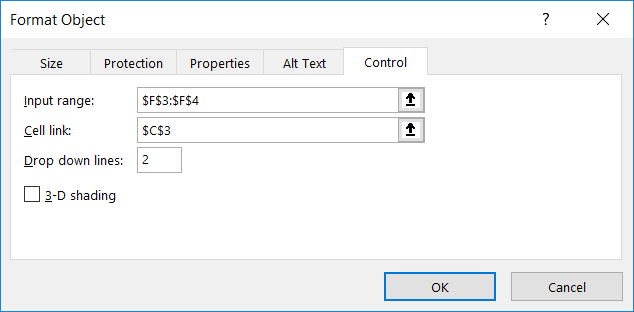Click OK and now the combo box is there and working.

## Changing Payoff Formula to Reflect Combo Box

If needed, you can move the combo by right-clicking it and dragging it to a new location. If you try that and reveal the content of cell C3, you will see that its value is 1 when the combo selection is "Call" and 2 for "Put" (this is because in our input range – cells F3-F4 – call is the first row and put is the second).

We must therefore change the condition in the IF function in our payoff calculation and replace the "Call" text (including the quotes) with the number 1 (no quotes). The existing formula in cell C8:

``=MAX(IF(C3="Call",C6-C4,C4-C6),0)-C5``

... becomes:

``=MAX(IF(C3=1,C6-C4,C4-C6),0)-C5``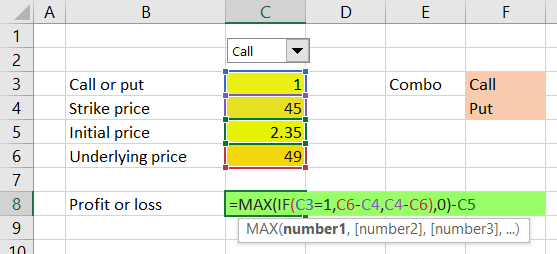What it does: If cell C3 value is 1 (which is when "Call" is selected in the combo box), it calculates call option payoff. If cell C3 value is anything else (which is when "Put" is selected and cell C3 value is 2), it calculates put option payoff.

Now we have a working profit or loss calculator for a call or put option, based on our selection in the combo box. Besides eliminating the risk of confusion and errors, the combo box is also much faster to switch between "Call" and "Put" compared to typing the words.

## Next Steps

Now we are going to make the calculator also work with short calls and short puts, and with positions involving multiple contracts.

Go to next part: Short Option Payoff and Position Size

By remaining on this website or using its content, you confirm that you have read and agree with the Terms of Use Agreement.

We are not liable for any damages resulting from using this website. Any information may be inaccurate or incomplete. See full Limitation of Liability.

Content may include affiliate links, which means we may earn commission if you buy on the linked website. See full Affiliate and Referral Disclosure.

We use cookies and similar technology to improve user experience and analyze traffic. See full Cookie Policy.